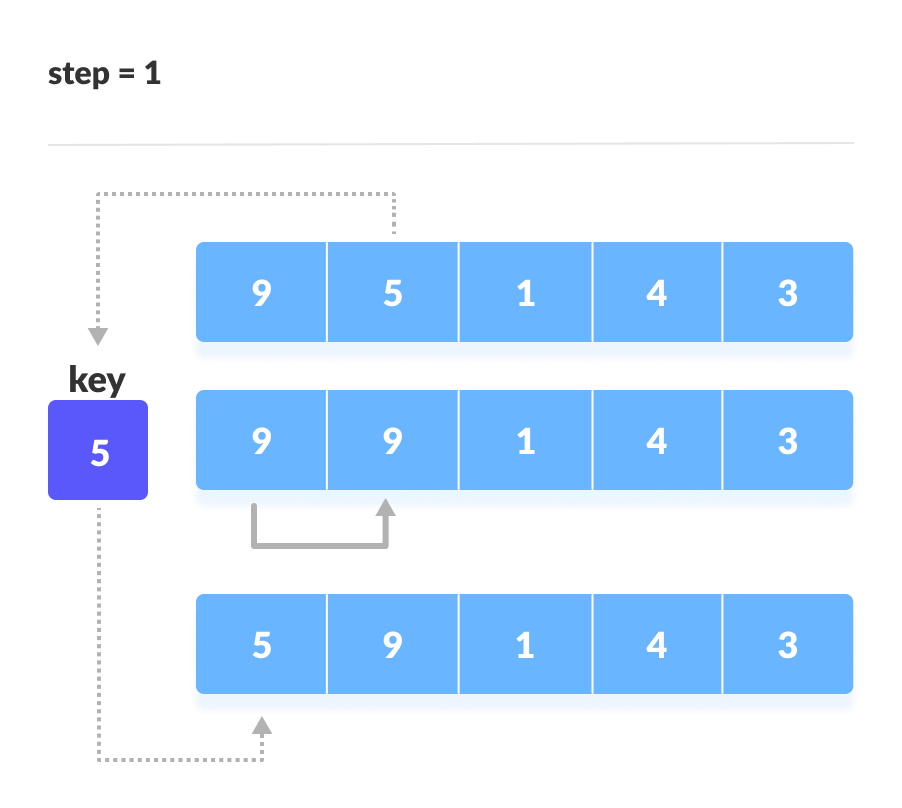# Insertion Sort Algorithm

#### In this tutorial, you will learn how insertion sort works. Also, you will find working examples of insertion sort in Python.

Insertion sort works similarly as we sort cards in our hand in a card game.

We assume that the first card is already sorted then, we select an unsorted card. If the unsorted card is greater than the card in hand, it is placed on the right otherwise, to the left. In the same way, other unsorted cards are taken and put at their right place.

A similar approach is used by insertion sort.

Insertion sort is a sorting algorithm that places an unsorted element at its suitable place in each iteration.

## How Insertion Sort Works?

Suppose we need to sort the following array.

1. The first element in the array is assumed to be sorted. Take the second element and store it separately in `key`.Compare `key` with the first element. If the first element is greater than `key`, then key is placed in front of the first element.If the first element is greater than key, then key is placed in front of the first element.
2. Now, the first two elements are sorted.Take the third element and compare it with the elements on the left of it. Placed it just behind the element smaller than it. If there is no element smaller than it, then place it at the beginning of the array.
3. Similarly, place every unsorted element at its correct position.

## Insertion Sort Algorithm

```insertionSort(array)
mark first element as sorted
for each unsorted element X
'extract' the element X
for j <- lastSortedIndex down to 0
if current element j > X
move sorted element to the right by 1
break loop and insert X here
end insertionSort```

## Python Examples

``````/* Insertion sort in Python */

def insertionSort(array):

for step in range(1, len(array)):
key = array[step]
j = step - 1

/* Compare key with each element on the left of it until an element smaller than it is found
For descending order, change key<array[j] to key>array[j].        */
while j >= 0 and key < array[j]:
array[j + 1] = array[j]
j = j - 1

/* Place key at after the element just smaller than it. */
array[j + 1] = key

data = [9, 5, 1, 4, 3]
insertionSort(data)
print('Sorted Array in Ascending Order:')
print(data)``````

## Complexity

Time Complexities

• Worst Case Complexity: `O(n2)`
Suppose, an array is in ascending order, and you want to sort it in descending order. In this case, worst case complexity occurs.Each element has to be compared with each of the other elements so, for every nth element, `(n-1)` number of comparisons are made.

Thus, the total number of comparisons = `n*(n-1) ~ n2`

• Best Case Complexity: `O(n)`
When the array is already sorted, the outer loop runs for `n` number of times whereas the inner loop does not run at all. So, there are only `n` number of comparisons. Thus, complexity is linear.
• Average Case Complexity: `O(n2)`
It occurs when the elements of an array are in jumbled order (neither ascending nor descending).

Space Complexity

Space complexity is `O(1)` because an extra variable `key` is used.

## Insertion Sort Applications

The insertion sort is used when:

• the array is has a small number of elements
• there are only a few elements left to be sorted

# Special 95% discount

## 2000+ Applied Machine Learning & Data Science Recipes

### Portfolio Projects for Aspiring Data Scientists: Tabular Text & Image Data Analytics as well as Time Series Forecasting in Python & R## Two Machine Learning Fields

There are two sides to machine learning:

• Practical Machine Learning:This is about querying databases, cleaning data, writing scripts to transform data and gluing algorithm and libraries together and writing custom code to squeeze reliable answers from data to satisfy difficult and ill defined questions. It’s the mess of reality.
• Theoretical Machine Learning: This is about math and abstraction and idealized scenarios and limits and beauty and informing what is possible. It is a whole lot neater and cleaner and removed from the mess of reality.

Data Science Resources: Data Science Recipes and Applied Machine Learning Recipes

Introduction to Applied Machine Learning & Data Science for Beginners, Business Analysts, Students, Researchers and Freelancers with Python & R Codes @ Western Australian Center for Applied Machine Learning & Data Science (WACAMLDS) !!!

Latest end-to-end Learn by Coding Recipes in Project-Based Learning:

Applied Statistics with R for Beginners and Business Professionals

Data Science and Machine Learning Projects in Python: Tabular Data Analytics

Data Science and Machine Learning Projects in R: Tabular Data Analytics

Python Machine Learning & Data Science Recipes: Learn by Coding

R Machine Learning & Data Science Recipes: Learn by Coding

Comparing Different Machine Learning Algorithms in Python for Classification (FREE)

`Disclaimer: The information and code presented within this recipe/tutorial is only for educational and coaching purposes for beginners and developers. Anyone can practice and apply the recipe/tutorial presented here, but the reader is taking full responsibility for his/her actions. The author (content curator) of this recipe (code / program) has made every effort to ensure the accuracy of the information was correct at time of publication. The author (content curator) does not assume and hereby disclaims any liability to any party for any loss, damage, or disruption caused by errors or omissions, whether such errors or omissions result from accident, negligence, or any other cause. The information presented here could also be found in public knowledge domains.  `